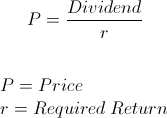# PV of Stock - Zero Growth

PV of Stock - No Growth Calculator (Click Here or Scroll Down)The formula for the present value of a stock with zero growth is dividends per period divided by the required return per period. The present value of stock formulas are not to be considered an exact or guaranteed approach to valuing a stock but is a more theoretical approach.

The present value of a stock formula used above is specific to stocks that have zero growth, or no growth. It is important to remember that the period used for both dividends and the required return must match. For example, if one is using annual dividends, then the annual return must be used.

## Use of the PV of a Stock with Zero Growth

As stated above, the present value of a stock with no growth formula is more conceptual than forcefully implemented in every circumstance. The general idea is that a stock is essentially like any other form of investment and should be valued based on future cash flows. In practice, investors and analysts may take various factors besides only dividends into consideration. Examples of other considerations may be expected future earnings based on news, appreciation of a stock due to retained earnings, and overall economy (inflation, production, and capital). Also, there are various methods or models used in trying to value a stock.

## How is the PV of a Stock with Zero Growth Derived?

The present value of a stock is broadly considered the sum of the discounted future cash flows. Dividends are considered the future cash flows as the appreciation of a stock is not realized unless sold. Since the stock is held with no maturity date, one could consider a stock to be a perpetuity, in that its dividends are to be received infinitely.

The formula for the present value of a stock with no growth shown at the top of the page theorizes that the stock is a perpetuity where dividends will be received on an ongoing basis for an unending period of time. Dividends would be denoted as cash flows in the perpetuity formula.

New to Finance?